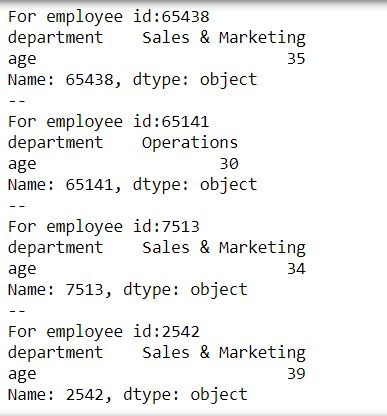Search
•Ekta Aggarwal

# iterrows for dataframes in Python

Let us consider this csv file:

HR_data
.csv

`import pandas as pd`

Let us firstly read and save our csv file and define index_col = 0, telling Python that 1st column is the row names.

```hrdata = pd.read_csv("HR_data.csv",index_col = 0)

Let us firstly see what happens if we iterate over hrdata?

```for i in hrdata:
print(i)```

It returns the column names:To iterate over various rows and column entries in a data frame we use iterrows function

Here we have two iterator variables: row and col

and our iterable object is hrdata.iterrows( )

```for row,col in hrdata.iterrows():
print("For employee id:" + str(row))
print(col)
print('--')```

Here row is taking the row name (our employee id)

while col is denoting the values for each row-col combinationTask: Fetch the row and column entries only for department column using iterrows

To achieve this we have filtered our iterator col by 'department'

```for row,col in hrdata.iterrows():
print("For employee id:" + str(row))
print(col['department'])
print('--')```Task: Fetch the row and column entries only for department and age columns using iterrows

To achieve this we have filtered our iterator col by providing a list ['department','age']

```for row,col in hrdata.iterrows():
print("For employee id:" + str(row))
print(col[['department','age']])
print('--')```See All